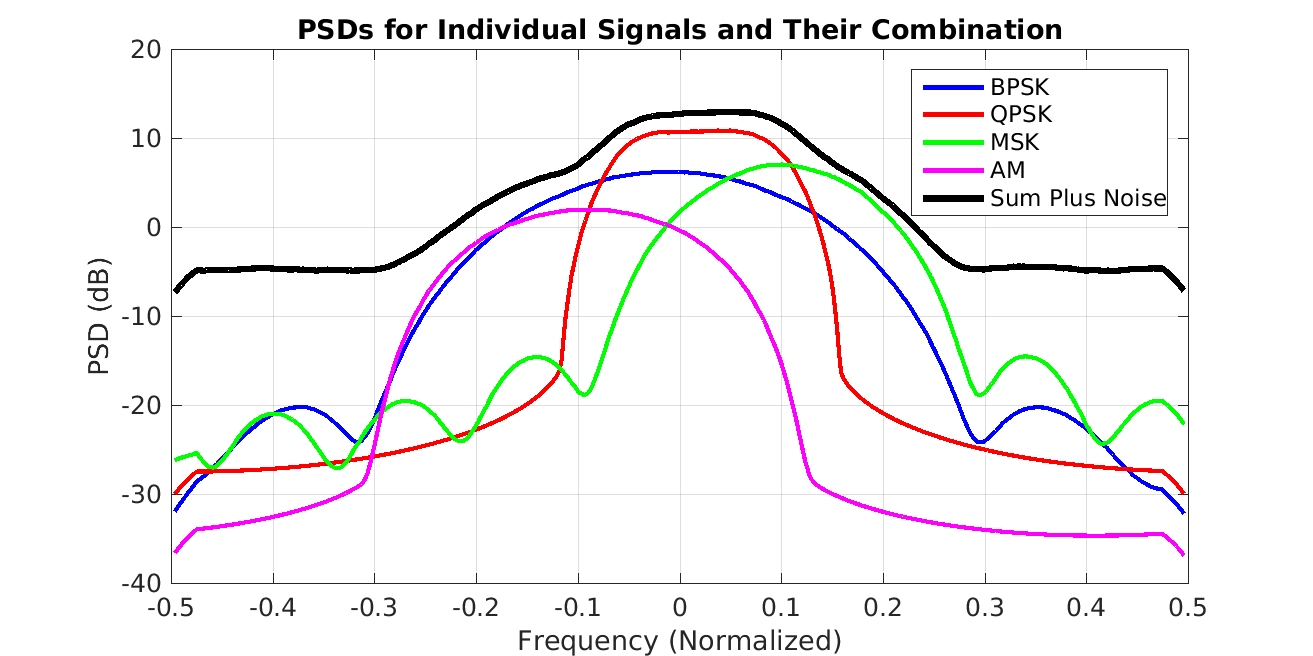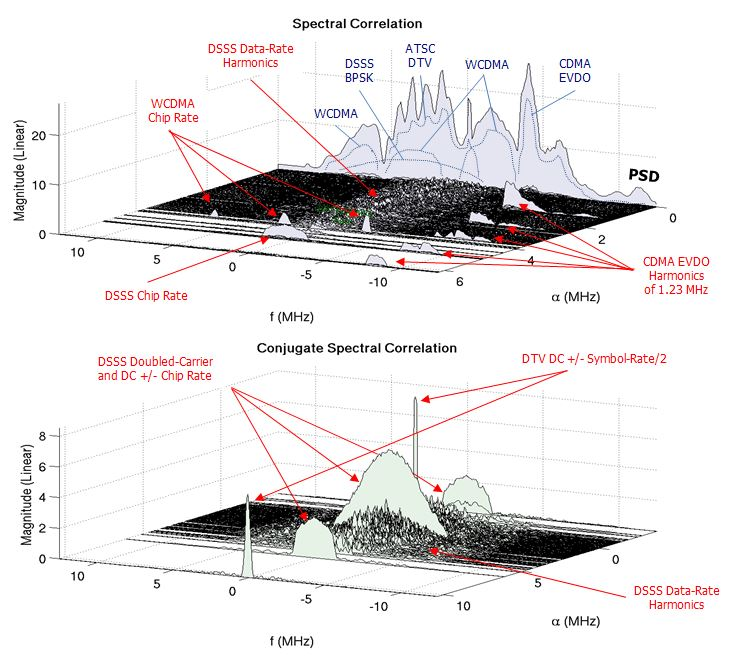# Signal Selectivity

We can estimate the spectral correlation function of one signal in the presence of another with complete temporal and spectral overlap provided the signal has a unique cycle frequency.

In this post I describe and illustrate the most important property of cyclostationary statistics: signal selectivity. The idea is that the cyclostationary parameters for a single signal can be estimated for that signal even when it is corrupted by strong noise and cochannel interferers. ‘Cochannel’ means that the interferer occupies a frequency band that partially or completely overlaps the frequency band for the signal of interest.

A mixture of received RF signals, whether cochannel or not, is accurately modeled by the simple sum of the signals, as in$x(t) = s_1(t) + s_2(t) + \ldots + s_K(t) + w(t), \hfill (1)$

where$w(t)$ is additive noise. We can write this more compactly as$x(t) = \displaystyle \sum_{k=1}^K s_k(t) + w(t). \hfill (2)$

A noisy cochannel mixture of four signals is shown here:Figure 1. Illustration of a three-signal cochannel situation. A receiver can only sense the sum of the signals, producing the power spectrum shown by the black line. A goal of CSP is to process that sensed data and determine the number of individual signals that make it up, and also their modulation types and parameters.

If the$K$ involved signals are mutually independent (or at least uncorrelated), and are also independent from the noise$w(t)$, then it is easy to show that the spectral correlation function for the sum$x(t)$ is equal to the sum of the spectral correlation functions for each of the involved signals,$S_x^\alpha(f) = \displaystyle \sum_{k=1}^K S_{s_k}^\alpha(f) + S_w^\alpha(f). \hfill (3)$

When the non-conjugate spectral correlation function is considered, and the cycle frequency$\alpha = 0$, then the spectral correlation function is identical to the power spectrum, and we have$S_x^0(f) = \displaystyle \sum_{k=1}^K S_{s_k}^0 (f) + S_w^0(f), \hfill (4)$

or in conventional notation,$S_x(f) = \displaystyle \sum_{k=1}^K S_{s_k} (f) + S_w(f). \hfill (5)$

Now each signal has some finite power, and so has a non-zero power spectrum, including the noise signal. So the power spectrum for the sum of the signals is equal to the sum of the power spectra. When the signals are cochannel, the individual power spectra add together across a common band of frequencies, and we end up getting a mixture that cannot be unmixed. That is, the individual power spectra cannot be obtained from the sum-signal power spectrum without substantial prior information. On the other hand, if the signals were not cochannel, but instead occupied disjoint frequency bands, the power spectrum for the sum signal would still be additive, but the individual power spectra could be isolated by linear time-invariant filtering and each could then be associated with one or another of the component signals.

Now suppose the noise$w(t)$ is stationary, and choose a cycle frequency$\alpha \neq 0$. Then the spectral correlation for the sum signal is given by$S_x^\alpha (f) = \displaystyle \sum_{k=1}^K S_{s_k}^\alpha (f), \hfill (6)$

since the noise is not cyclostationary. This is the well-known noise tolerance of cyclostationary-signal parameters. In practice, we use estimates of the spectral correlation function, which are in fact influenced by the noise, but as the length of the processed data-block increases, the effect decreases in size.

On to signal selectivity. Suppose that one of the$K$ signals, say $s_j(t)$, possesses a cycle frequency$\alpha_*$ that is possessed by no other signal in the sum. Then,$S_x^{\alpha_*} (f) = S_{s_j}^{\alpha_*} (f). \hfill (7)$

Because we can operate on the sum data, yet obtain the spectral correlation for a selected signal, we say that the spectral correlation function is signal selective.

Consider the example shown above in Figure 1, where four signals are spectrally (and temporally) overlapped and added to noise. Blindly estimated spectral correlation functions for each of the individual signals are shown below, arranged around a plot of the blindly estimated spectral correlation function for the sum signal:Figure 2. Illustration of the feature separability in the f-alpha plane for the data in Figure 1. We can use CSP to form the estimates in the central black box. The spectral correlation functions for the individual signals are arrayed around the central box for reference.

The individual slices of the spectral correlation function are labeled so that the contribution to the sum-signal spectral correlation can be identified (by you, dear reader) for each signal in the sum.

The example above used only synthetic (simulated) signals and noise. To convince the reader that CSP and signal-selectivity work in the real world on real signals captured by real receivers (for real!), consider the following example, which is the result of processing a scene containing multiple captured signals added together:Figure 3. Illustration of the signal-selectivity property for an artificial combination of real-world captured data files.

### Why do we Care About Signal Selectivity?

One reason is that the separation of the features for the individual signals in the spectral correlation plane allows the possibility of jointly detecting and classifying all$K$ cochannel signals (My Papers [25,26]). A related reason is that signal selectivity enables detecting a particular signal of interest in spite of strong cochannel interference, which is not of interest.

Another reason is that signal selectivity allows the creation of various interference-tolerant parameter estimators, such as time-difference-of-arrival (TDOA) estimators, which use the data from two widely separated sensors to estimate the relative delay of a signal impinging upon both. Typical cross-correlation-based TDOA estimators are highly vulnerable to the presence of cochannel interference, but cyclic (cyclostationarity-exploiting) estimators are not (My Papers [2,4,21,23]).

In later posts, we’ll see that the signal-selectivity property of the spectral correlation function (and cyclic autocorrelation function) extends to higher-order statistics only if we use higher-order cyclic cumulants, but not if we use cyclic moments.I'm a signal processing researcher specializing in cyclostationary signal processing (CSP) for communication signals. I hope to use this blog to help others with their cyclo-projects and to learn more about how CSP is being used and extended worldwide.

## 7 thoughts on “Signal Selectivity”

1.Heba. says:

compact and clear.

2.Mohamed says:

That is a very good example, but I have a question about the noise because you assume its stationary.
In general, does the white Gaussian noise (with a flat PSD over a wide range of frequencies) have any cyclic frequencies? I mean does it cause any distortion in the cyclic spectrum when added to the signal?

1.Chad Spooner says:

Thanks. Yes, white Gaussian noise is stationary. And it is a good model for noise we typically encounter in our electronic devices like receivers. Adding WGN to the signal(s) does degrade the spectral correlation function estimate, but the ideal spectral correlation function (the limit spectral correlation function, which considers all time) is not affected. This just means that we can approach the ideal function with our estimate in the presence of arbitrary-strength WGN provided we can observe the data for a sufficiently long time.

1.Mohamed says:

How the noise will degrade the spectral correlation estimate? so it will not add any new cyclic frequencies but it will distort the SCF at the existing cyclic frequencies of the signal? or it will distort the SCF only at $\alpha$=0 (the PSD) ?
It would be great if you can refer to some equations that analyze this issue

1.Chad Spooner says:

The noise will affect measurements of all parts of the spectral correlation function. I tend to not use the word “distort” here, because I try to reserve that for things like convolution (for example, a propagation channel distorts the signal). But yeah, all parts of the SCF are affected to a greater or lesser degree. For insight into the degree, see the equations and discussion in the resolution product post.

1.Mohamed says:

The equations state that: $S_x^0(f) = S_s^0(f)+S_n^0(f)$ only for $\alpha=0$, and for $\alpha$ not equal zero the SCF will be $S_x(f) = S_s(f)$. So, how will the noise’s effect appear in the case of $alpha$ not equal zero?
2.Chad Spooner says:
If you want to show latex-formatted equations in a comment, try adding the word latex after the first $. As in$S_x^\alpha(f)$, which is S latex S_x^\alpha (f) S, where the ‘S’ represent ‘$’.